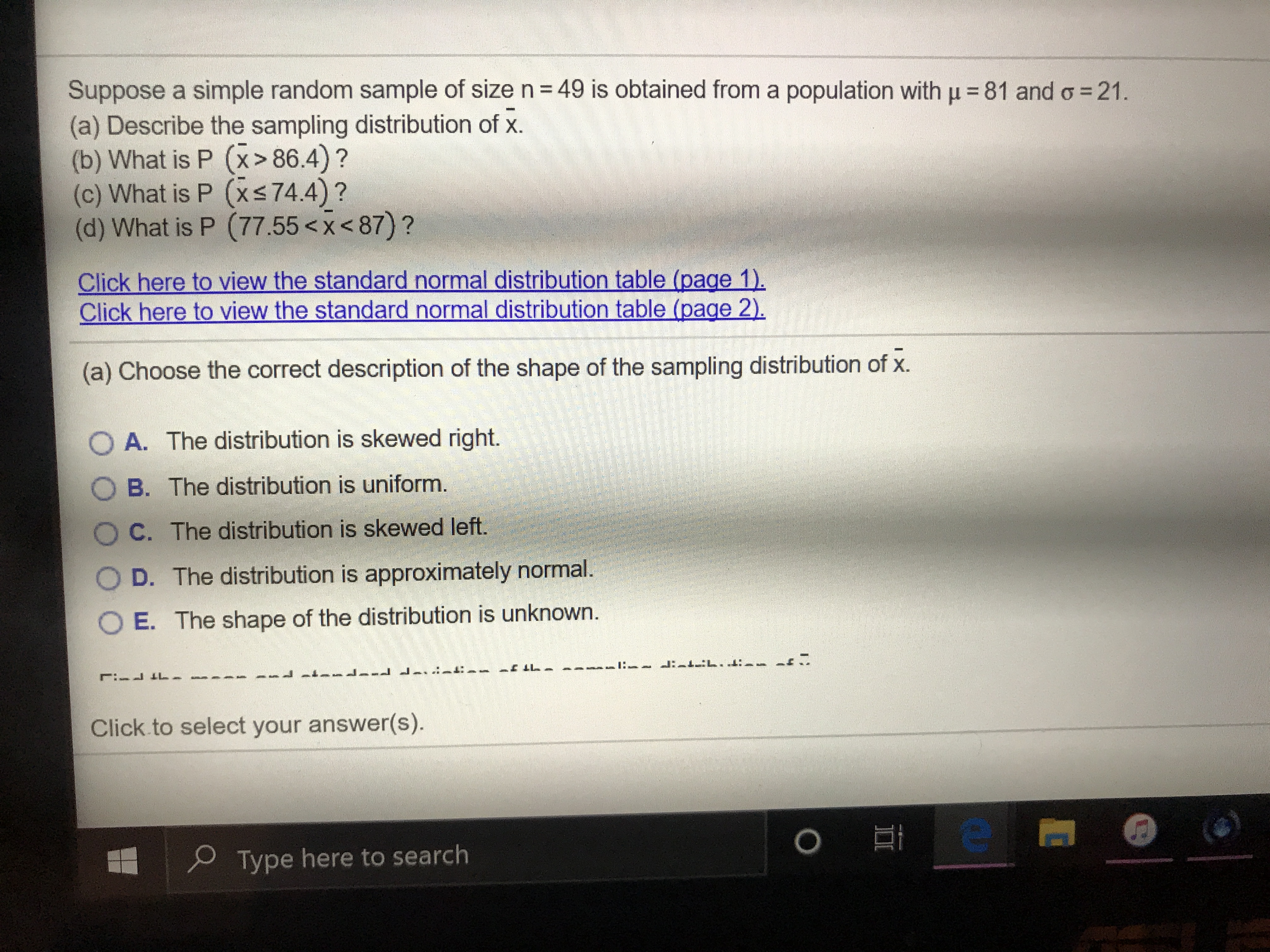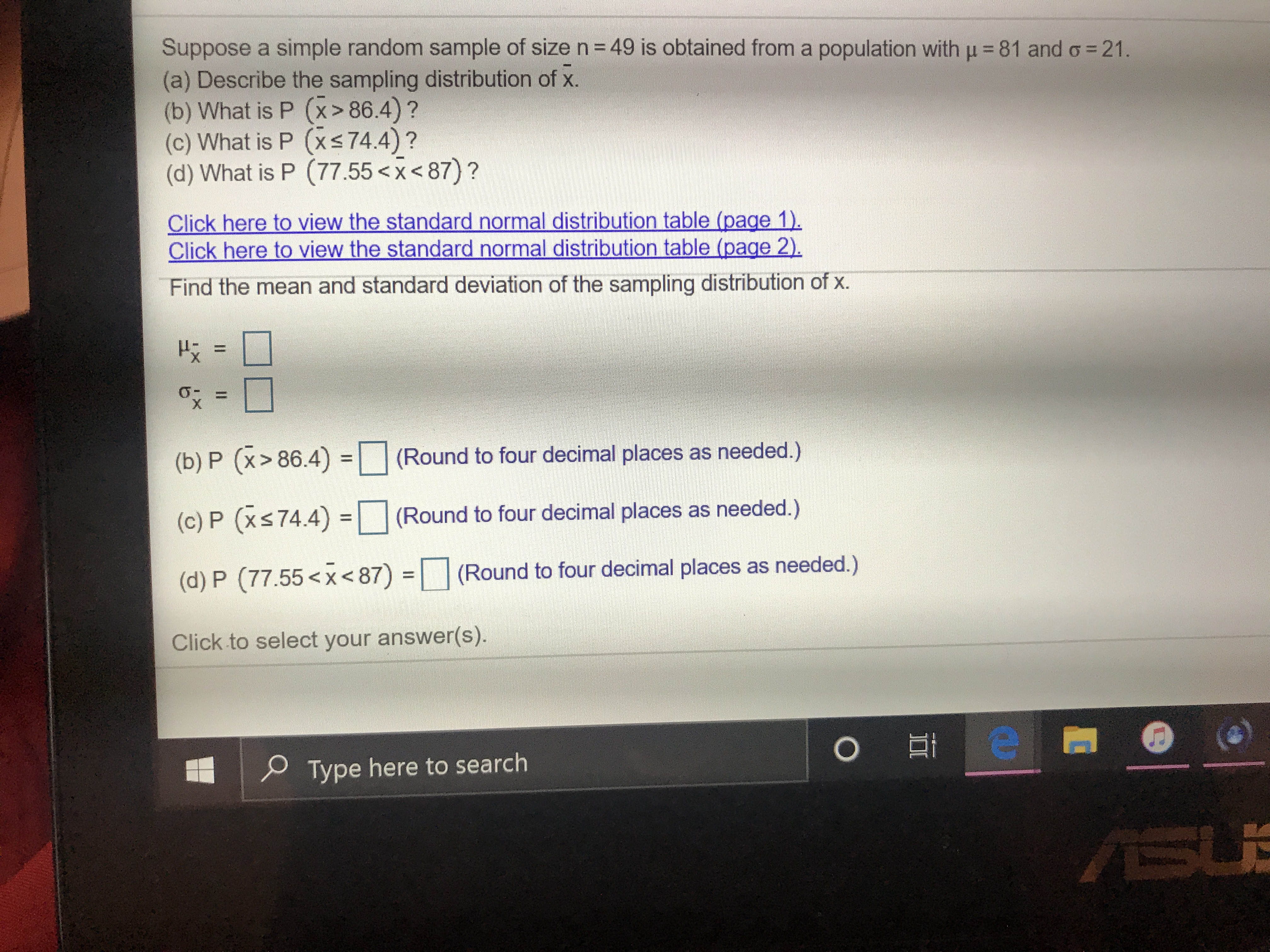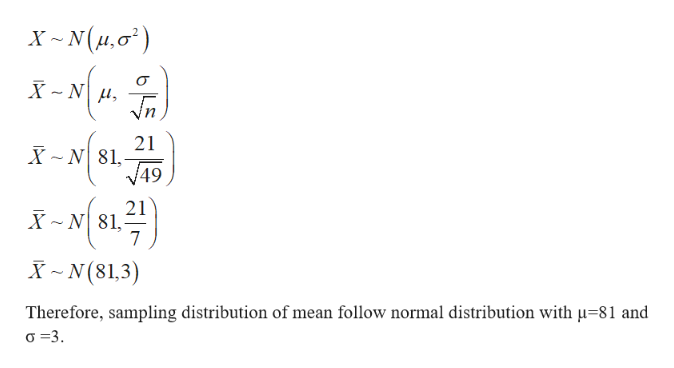# Suppose a simple random sample of size n= 49 is obtained from a population with u = 81 and o = 21.(a) Describe the sampling distribution of x.(b) What is P (x> 86.4)?(c) What is P (xs74.4)?(d) What is P (77.55 86.4)?(c) What is P (xs74.4)?(d) What is P (77.55 86.4) =(Round to four decimal places as needed.)(c) P (xs74.4) =(Round to four decimal places as needed.)%3D(d) P (77.55

Question
39 viewshelp_outlineImage TranscriptioncloseSuppose a simple random sample of size n= 49 is obtained from a population with u = 81 and o = 21. (a) Describe the sampling distribution of x. (b) What is P (x> 86.4)? (c) What is P (xs74.4)? (d) What is P (77.55help_outlineImage TranscriptioncloseII Suppose a simple random sample of size n= 49 is obtained from a population with u = 81 and o = 21. (a) Describe the sampling distribution of x. (b) What is P (x> 86.4)? (c) What is P (xs74.4)? (d) What is P (77.55 86.4) = (Round to four decimal places as needed.) (c) P (xs74.4) = (Round to four decimal places as needed.) %3D (d) P (77.55
check_circle

Step 1

According to the provided information,

Step 2

A)

The sampling distribution of mean can be calculated as:help_outlineImage TranscriptioncloseX ~ N(µ,0°) X - N H, In 21 X ~ N 81,- V49 21 81,- X ~ N(81,3) Therefore, sampling distribution of mean follow normal distribution with µ=81 and o =3. fullscreen
Step 3

B)

The required probability ca...

### Want to see the full answer?

See Solution

#### Want to see this answer and more?

Solutions are written by subject experts who are available 24/7. Questions are typically answered within 1 hour.*

See Solution
*Response times may vary by subject and question.
Tagged in
MathStatistics

### Other